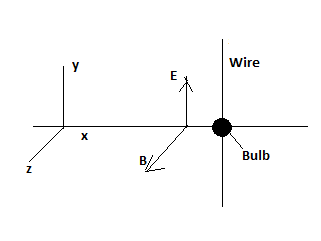# A long, thin steel wire is cut in half and each half is connected to a different terminal of a...

## Question:

A long, thin steel wire is cut in half and each half is connected to a different terminal of a light bulb. An electromagnetic (EM) plane wave with {eq}\vec{E}(x,y,z,t)=E_0sin(kx-wt) \hat{ y}, \vec{B}(x,y,z,t) = B_0sin(kx-wt) \hat{z} {/eq} moves past the wire, as shown. In which direction is the wave propagating? Explain your reasoning. Suppose the wire were oriented parallel to the y-axis, as shown. Would the bulb glow in this case? Explain## Electromagnetic Wave and EMF Induced on a Conductor

An electromagnetic wave consists of mutually perpendicular electric field and magnetic field oscillations. As the wave is a transverse one there is no oscillations in the direction of propagation. According to Lorentz equation a charge q moving with a velocity v will experience a magnetic force {eq}F = q v B \sin \theta {/eq} in a magnetic field B. Here {eq}\theta {/eq} is the angle between the direction of the velocity and magnetic field. Large number of free electrons are available in conductors. So if a conductor is moved in a normal magnetic field, the electrons in the conductor will experience Lorentz force and electrons will drift to on end of the conductor to develop a potential difference across the conductor given by the equation {eq}E = B L v {/eq}. Here L is the length of the conductor.

Given data

• The magnetic field and electric field in the plane electromagnetic wave is given as {eq}B ( x, y,z, t) = B_0 \sin ( kx - \omega t ) \hat z \\ E ( x, y, z, t ) = E_0 \sin ( kx - \omega t ) \ \hat y {/eq}
• Two pieces of steel wire connected to the terminals of the bulb is kept parallel to the y axis as shown in the figure.

Part a)

The negative sign before the angular frequency {eq}\omega {/eq} term indicates that the wave is propagating in the positive x direction.

Therefore the electromagnetic wave is propagating in the positive x direction.

Moreover the electric field and magnetic field oscillations have no component in the x direction but they have components in the y and z direction. This also helps us to conclude that the wave is propagating parallel to the x axis.

Part b)

The bulb will glow.

When the wire is oriented parallel to the y axis, the magnetic field oscillations are perpendicular to the length of the wire. As the wave pass by the conductor, the electrons in the conductor will experience magnetic force and it will drift to one end a create a potential difference.

Because the passing of the wave is equal to the moving of the conductor in a perpendicular magnetic field.

Since the magnetic field is oscillating in the positive and negative directions along the z axis the EMF induced will be an alternating one.

Due to this EMF the bulb will glow. The working of the conductor is just similar to that of wire antenna.